# Task Allocation Model for Optimal System Cost Using Fuzzy C-Means Clustering Technique in Distributed System

Task Allocation Model for Optimal System Cost Using Fuzzy C-Means Clustering Technique in Distributed System
Department of Mathematics, DIT University, Dehradun 248009, India

CBRI-IIT Roorkee, Roorkee 247667, India

Corresponding Author Email:
Page:
59-68
|
DOI:
https://doi.org/10.18280/isi.250108
8 October 2019
|
Accepted:
10 December 2019
|
Published:
29 February 2020
| Citation

OPEN ACCESS

Abstract:

The task scheduling is an important activity in distributed system environment to divide the proper load among the available processors. The requirement of efficient task scheduling technique is an important issue in distributed computing systems, which can balance the load in such a way, so that no processor remains idle. Further, it can provide proper utilization of available resources and minimize the response time and system cost, with the maximum system reliability. In this paper the novel task allocation technique is being proposed with the aim of minimizing the response time and system cost. The method of clustering is used for the proper distribution of tasks on the processors. The proposed technique uses Fuzzy C-Means clustering technique and Hungarian method for task allocations. The performance of the algorithm is evaluated through examples and the results are compared with some existing models.

Keywords:

1. Introduction

With advance computational technologies and high-speed networks, distributed computing system (DCS) has become popular worldwide. Distributed computing system has multiple processors located at geographically distant places i.e. at different cities or countries, interconnected by communication links. There are many factors which considerably affects the performance of the DCS viz. speed of processors, memories, failure rate of processors, failure rate of interconnecting network etc. One such & highly considerable factor is allocation of modules to processors. This allocation should be in such a way that system cost is minimized with some average load on each processor, so that no processor remains idle. Also, the available resources should be utilized to its maximum. Task allocation can be done in two ways:

1. Static Allocation- when a module is assigned to a processor, it remains with the processor till the completion of the process.

2. Dynamic Allocation- a module when allocated to one processor may migrate to another processor according to requirement of the system.

Dynamic allocation uses current state information of the system in making decision while static allocation using Random or Round Robin don’t use any information of current state of nodes for load balancing [1-4]. Different algorithms for module allocation are proposed with different objectives. Some have objective of balancing the load [2, 4] while some an objective of minimizing response time and maximizing system reliability [5-9]. Topcuoglu et al.  discussed and proposed two novel scheduling algorithms, the Heterogeneous Earliest-Finish-Time (HEFT) algorithm and the Critical-Path-on-a-Processor (CPOP) algorithm, for a bounded number of heterogeneous processors with an objective to meet high performance and fast scheduling time simultaneously. Falta et al.  propose a fully distributed K-Means algorithm (Epidemic K-Means) which does not require global communication and is intrinsically fault tolerant, which otherwise lacks in large scale systems and provides a clustering solution which can approximate the solution of an ideal centralized algorithm over the aggregated data as closely as desired. Rashidi  proposes an algorithm, based on multi-objective scheduling cuckoo optimization algorithm (MOSCOA), in which each cuckoo represents a scheduling solution in which the ordering of tasks and processors allocated to them are considered. In addition, the operators, of cuckoo optimization algorithm defined, are usable for scheduling scenario of the directed acyclic graph of the problem. Bahmani and Mueller  proposed a fast signature-based clustering algorithm that clusters processes exhibiting similar execution behavior. Vidyarthi and Tripathi  developed a heuristic approach, based on genetic algorithm, to find the near optimal solution.

In this paper, the proposed work uses Fuzzy C-Means (FCM) clustering algorithm to allocate task to different processors with the objective of minimizing system cost and response time. It is different from other clustering techniques in such a way that the data point is not a member of only one cluster, but may belong to more clusters with certain degree of membership value. If the data points are located on the boundaries of the clusters, they are not forced to belong to a certain cluster and thus have flexibility of being the member of others clusters too, for better performance of system. FCM is an iterative process and it stops when the objective function acquires desired degree of accuracy. The performance of the proposed algorithm is illustrated with examples. The outcomes are compared with some existing models. The road map of the paper is as follows- section 2 describes the problem statement. Section 3, illustrates the preliminaries for the proposed technique. Section 4, proposes the algorithm. Section 5, describes the performance evaluation and comparisons with existing works and at last section 6 draws the conclusion.

2. Problem Statement

The problem addressed in the paper is concerned with allocation of tasks to processors of a distributed system with the goal of minimizing response time and system cost. The distributed system consists of multiple processors, where multiple users can work simultaneously from different sites. The processors available, at different sites in the system, process the requests according to availability. Each processor has its own computation capacity and memory while communication network has a limited communication capacity. In real time scenario, some failure rate is also associated with each processor and communication link. Figure 1 shows a general model of distributed system.

## 1.png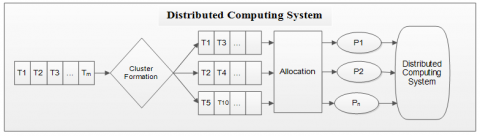Figure 1. Distributed system model

Different factors are considered while allocating tasks to processors. Two main factors are Execution Time of tasks at different processors  and Inter Processor Communication (IPC) overhead [14, 15]. A set of tasks, to be executed parallel, are to be allocated to n processors where 1£i£m, 1£k£n & m>n. The tasks require processor resources such as computational capacity and memory capacity. The system resources have restricted capacity and a failure rate is associated with each component. The purpose of task allocation is to find optimal allocation of each task to the processors such that the system cost and response time are minimized with proper mapping of tasks to processors so that no processor remains idle. Furthermore, the task requirements and resource limitations are met.

3. Preliminaries

3.1 Execution Time (ET)

The execution time, eik is the amount of time taken by task ti, which is to be executed on the processor pk, where 1£i£m, 1£k£n. If a task ti is assigned to a processor pk but is not executed due to absence of some resources, then eik of the task on the processor is taken to be ∞ i.e. very large value. The execution time, eik, of each task on each processor can be written in the form of Execution Time Matrix (ETM). The Total Execution Time (ET) is calculated as given :

$E T=\sum_{i=1}^{m} \sum_{k=1}^{n} e_{i k} x_{i k}$    (1)

x is an assignment matrix such that

$x_{i k}=\left\{\begin{array}{l}1, \text {if task } T_{i} \text { is assigned to processor } p_{k} \\ 0, \text { else }\end{array}\right.$

3.2 Inter Task Communication Time (ITCT)

The Inter Task Communication Time, cij, is the amount of time incurred due to the data units exchanged between the tasks ti and tj if they are executed on different processors. When some tasks are assigned to same processor, then cij=0. Total Inter-Task Communication Time (ITCT) of program is calculated by using Eq. (2) given as follows :

$I T C T=\sum_{i, j=1}^{m} \sum_{k, l=1 \atop k \neq l}^{n} c_{i j} x_{i k} x_{j l}$    (2)

x is an assignment matrix such that

$x_{i k}=\left\{\begin{array}{l}1, \text { if task } t_{i} \text { is assigned to processor } p_{k} \\ 0, \text { else }\end{array}\right.$

3.3 Response time (RT)

Response time of a system is the amount of time taken by each processor for the computation of the given tasks including inter task communication time. It is defined by considering the processor with heaviest aggregate computation and communication loads of the processor. Response time (RT) of a system is calculated as follows:

$R T=\max \left\{\sum_{i=1}^{m} \sum_{k=1}^{n} e_{i k} x_{i k}+\sum_{i, j=1}^{m} \sum_{l=1 \atop k \neq l}^{n} c_{i j} x_{i k} x_{j l}\right\}$    (3)

3.4 System Cost (SC)

The System Cost (SC) of the system is the sum of total execution time and total inter task communication time i.e.

$S C=\sum_{i=1}^{m} \sum_{k=1}^{n} e_{i k} x_{i k}+\sum_{i, j=1}^{m} \sum_{k, l=1 \atop k \neq 1}^{n} c_{i k} x_{i k} x_{j l}$    (4)

3.5 Allocation constraints

The allocation depends on tasks requirements and system resources. Some of the constraints are considered in the proposed algorithm and are as follows:

• Processor load constraints: For task assignment, the total processing load required by all tasks assigned to processor k must be less than or equal to available computational load of processor k. If Li denotes the processing load required by task i and if pk denotes available processing load of processor k, then the following inequality for each processor must hold:

$\sum_{i=1}^{m} L_{i} x_{i k} \leq P_{k}$    (5)

$x_{i k}$ is an assignment matrix.

• Number of clusters: To execute a program parallel in minimum time, all the processors must be utilized wisely and tasks should be allocated in such a way that no processor remains idle. Keeping this point in mind the maximum number of clusters, a system can have, should be equal to number of processors i.e. neither should it exceed the number of processor nor should it be less than that else some of the processors may remain idle.
• Number of tasks in a processor: To execute a program parallel in minimum time and to balance load on all the processors, the maximum number of tasks in a cluster should be $\leq \frac{m}{n}$, where m is the number of tasks and n is the number of processors.
4. Proposed Work

In this section, first the Fuzzy C-Means clustering technique have been discussed and then explains how it may be employed for task allocation.

4.1 Fuzzy C-means clustering technique

Clustering groups the objects of similar nature and the metric is supposed to be defined on nature of addressed problem. Clustering can be hierarchical or partitioned. Hierarchical clustering is organized as tree, having a set of nested clusters, while partitioned clustering is division of objects into non-overlapping cluster in such a way that each object is contained exactly in one cluster. But, sometimes to improve and optimize the solution, it becomes an essential requirement to shift an object/s from one cluster to some other cluster by taking into consideration the parameters, constraints and available resources. Thus, having a flexibility of an object, of being a member of other clusters too, makes the system more efficient. Fuzzy C-Means clustering provides this flexibility to the objects where data objects (points) are grouped into overlapping clusters. It is different from other techniques in a way that in this technique the data point can potentially belongs to multiple clusters with a variable degree of membership value in each cluster. So, if data points are located on the boundaries of the clusters, they are not forced to belong to a certain cluster and have flexibility of being the member of others clusters too, for better performance of system. Clusters are formed according to distance, between data points and cluster centers, which characterized by membership values of data points for different clusters. Larger distance of data point from cluster centre is characterized by smaller membership value and smaller distance of is characterized by larger membership value. Fuzzy C-Means (FCM) is an iterative process and it stops when the objective function acquires desired degree of accuracy.

This clustering is based on Zadeh’s idea of fuzzy which was introduced on 1965. This algorithm does not classify fuzzy data, it classifies crisp data into fuzzy clusters. Fuzzy C-Means clustering technique can be summarized as below:

1. Generate n clusters randomly
2. Cluster centroids are calculated.
3. Finding Euclidean distance of each data point from each cluster centre.
4. Finding the membership value of each data point for each cluster, with the help of Euclidean distance.
5. Updating the clusters by taking membership value into consideration.
6. Computing new cluster centroid based on updated clusters.
7. Repeating the steps b) to f) until there is no change in the cluster centre or the difference of membership value is equal to the desired degree of accuracy.

4.2 Proposed algorithm

4.2.1 Fetch the data set

Fetch the data set. Inputs are:

1. A program of m tasks i.e. T={t1,t2,t3,…,tm}.
2. A set of n processors i.e. P={p1,p2,p3,…,pm}.
3. A set of n clusters i.e. G={g1,g2,g3,…,gm}.
4. ET(eik) and ITCT(cij) are taken in the form of matrices as Execution Time Matrix (ETM) and Inter Task Communication Time Matrix (ITCTM).

4.2.2 Fuzzy C-means clustering technique to form clusters

Let G denotes the clusters and T denotes the tasks, then form a matrix U of order G´T. Initializing Fuzzy C-Means (FCM) clustering technique by either forming the clusters randomly or using K-means clustering. In the clusters by Fuzzy C-means, the elements (i.e. tasks) belonging to one cluster may be shifted to another to balance the load and minimize the system cost, if required.

4.2.3 Assignment of tasks using Hungarian method

After forming clusters, the execution time (for each processor) and inter task communication time of each cluster is calculated. Then applying Hungarian method to allocate clusters to different processors in such a way that processor executes the clustered tasks in minimum time. If there is tie between two or more clustered tasks, the same above mentioned method can be used for allocation by using that combination which optimizes the system cost and response time.

4.2.4 Determination of Process Response Time (PRT)

The Process Response Time (PRT) is calculated using Eq. (6) as follows:

\begin{aligned} P R T_{k} &=\min \left\{\left(E T_{i 1}+I T C T_{i 1}\right),\left(\left(E T_{i 2}\right.\right.\right.\\+&\left.\left.I T C T_{i 2}\right), \ldots \ldots \ldots . .\left(E T_{i m}+I T C T_{i m}\right)\right\} \end{aligned}    (6)

Clustered Task giÎG is assigned to that processor for which PRT, i.e. (ETik+ITCTij), is minimum. This process is continued until all the clusters, gkÎG"1£k£n are assigned to all the processors.

4.2.5 Determination of Overall Process Response Time (OPRT) & System Cost (SC)

When the procedure of assigning the clustered tasks to different processors gets over, the OPRT for the distribution is the maximum of Process Response Time i.e.

$O P R T=\max \left\{P R T_{k}\right\} ; \forall 1 \leq k \leq n$    (7)

The System Cost (SC) after assigning all clustered tasks is calculated using Eq. (8) as follows:

$S C=\sum_{k=1}^{n} \operatorname{PRT}_{k}$    (8)

Flow Chart of the algorithm is shown in Figure 2.

## 2.png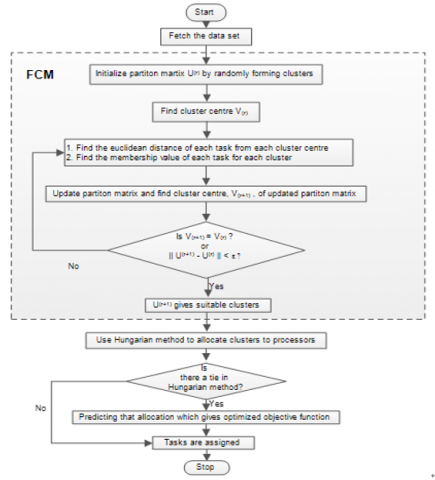Figure 2. Flow chart of proposed algorithm

5. Performance Analysis and Discussion

This section illustrates the proposed algorithm with the help of examples.

Table 1. Execution time matrix

 Processor → Tasks ↓ $p_1$ $p_2$ $p_3$ $t_1$ 174 176 110 $t_2$ 95 15 134 $t_3$ 196 79 156 $t_4$ 148 215 143 $t_5$ 44 234 122 $t_6$ 241 225 27 $t_7$ 12 28 192 $t_8$ 215 13 122 $t_9$ 211 11 208

Example 1: Consider a program made up of nine tasks $\left\{t_{1}, t_{2}, t_{3}, \dots . t_{9}\right\}$ to be allocated to three processors $\left\{p_{1}, p_{2}, p_{3}\right\}$. The execution cost of each task on each processor and the inter - task communication cost between tasks is considered in the form of matrices as given in Table 1 above and Table 2 below.

Table 2. Inter – task communication time matrix

 Tasks → ↓ $t_1$ $t_2$ $t_3$ $t_4$ $t_5$ $t_6$ $t_7$ $t_8$ $t_9$ $t_1$ 0 8 10 4 0 3 4 0 0 $t_2$ 8 0 7 0 0 0 0 3 0 $t_3$ 10 7 0 1 0 0 0 0 0 $t_4$ 4 0 1 0 6 0 0 8 0 $t_5$ 0 0 0 6 0 0 0 12 0 $t_6$ 3 0 0 0 0 0 0 0 12 $t_7$ 4 0 0 0 0 0 0 3 10 $t_8$ 0 3 0 8 12 0 3 0 5 $t_9$ 0 0 0 0 0 12 10 5 0

While using Fuzzy C – Means clustering technique, the partition matrix at each iteration (showing membership values of each task in each cluster) and the matrix of cluster centres are shown in Table 3 and Table 4.

Table 3. Iterations of partition matrix (showing membership values)

 U1 $g_1$ 0.26944 0.2953 0.69726 0.11647 0.2157 0.24482 0.34089 0.40289 0.25013 $g_2$ 0.65614 0.05707 0.05908 0.83766 0.6651 0.6326 0.14511 0.07719 0.05999 $g_3$ 0.07442 0.64763 0.24366 0.04587 0.1192 0.12258 0.514 0.51992 0.68988 U2 $g_1$ 0.13282 0.25301 0.82182 0.05699 0.19409 0.23627 0.32528 0.46318 0.35981 $g_2$ 0.81396 0.05594 0.03873 0.91363 0.66779 0.62349 0.15925 0.07537 0.07084 $g_3$ 0.05322 0.69105 0.13945 0.02938 0.13812 0.14024 0.51547 0.46145 0.56935 U3 $g_1$ 0.09418 0.1558 0.91397 0.04361 0.17362 0.22585 0.27523 0.59509 0.51028 $g_2$ 0.85947 0.03807 0.02343 0.92942 0.67249 0.63271 0.14793 0.07202 0.075 $g_3$ 0.04635 0.80613 0.0626 0.02697 0.15389 0.14144 0.57684 0.33289 0.41472 U4 $g_1$ 0.07885 0.04934 0.94406 0.03894 0.1554 0.21915 0.18803 0.74374 0.67206 $g_2$ 0.87947 0.01416 0.02079 0.93429 0.67186 0.64786 0.11284 0.06565 0.0748 $g_3$ 0.04168 0.9365 0.03515 0.02677 0.17274 0.13299 0.69913 0.19061 0.25314 U5 $g_1$ 0.06728 0.06102 0.91672 0.0361 0.14294 0.20859 0.12142 0.83341 0.77084 $g_2$ 0.8959 0.01931 0.03803 0.9374 0.67022 0.66874 0.07791 0.05469 0.06697 $g_3$ 0.03682 0.91967 0.04525 0.0265 0.18684 0.12267 0.80067 0.1119 0.16219 U6 $g_1$ 0.05997 0.10523 0.88754 0.03562 0.13794 0.19827 0.08842 0.86924 0.8124 $g_2$ 0.90628 0.03409 0.05572 0.93707 0.6669 0.6861 0.05756 0.04801 0.06017 $g_3$ 0.03375 0.86068 0.05674 0.02731 0.19516 0.11563 0.85402 0.08275 0.12743 U7 $g_1$ 0.05587 0.13943 0.87322 0.03631 0.13676 0.19187 0.07063 0.88326 0.83039 $g_2$ 0.9125 0.04515 0.06542 0.93536 0.66271 0.69707 0.04585 0.0455 0.05691 $g_3$ 0.03163 0.81542 0.06136 0.02833 0.20053 0.11106 0.88352 0.07124 0.1127 U8 $g_1$ 0.05353 0.16252 0.86763 0.03715 0.13692 0.18832 0.06047 0.88926 0.8387 $g_2$ 0.9163 0.05237 0.06996 0.93375 0.65916 0.70357 0.03904 0.0447 0.05559 $g_3$ 0.03017 0.78511 0.06241 0.0291 0.20392 0.10811 0.90049 0.06604 0.10571 U9 $g_1$ 0.0522 0.17753 0.86573 0.03783 0.13737 0.18637 0.0545 0.89213 0.84274 $g_2$ 0.9186 0.05692 0.07202 0.93258 0.65665 0.70738 0.03501 0.04448 0.05511 $g_3$ 0.0292 0.76555 0.06225 0.02959 0.20598 0.10625 0.91049 0.06339 0.10215 U10 $g_1$ 0.05144 0.18711 0.86516 0.0383 0.13778 0.18529 0.05091 0.89362 0.84479 $g_2$ 0.91997 0.05975 0.07298 0.93181 0.65501 0.70963 0.03259 0.04444 0.05495 $g_3$ 0.02859 0.75314 0.06186 0.02989 0.20721 0.10508 0.9165 0.06194 0.10026

Table 4. Iterations of cluster centres
 Iterations ↓ No. of Clusters Coordinates Iterations ↓ No. of Clusters Coordinates x y z x y z Center 1 g_1 155 90 133.33333 Center 6 g_1 203.98745 44.22856 156.54067 g_2 144.33333 224.66667 97.33333 g_2 153.95814 206.81627 109.35136 g_3 146 17.33333 174 g_3 64.68694 27.34281 157.40131 Center 2 g_1 166.38193 77.53152 144.41094 Center 7 g_1 204.94189 41.08747 157.21112 g_2 148.08071 209.34167 108.82608 g_2 155.18978 206.87612 108.50419 g_3 143.31387 23.10518 164.60876 g_3 57.98387 28.60875 160.8326 Center 3 g_1 177.83962 66.48551 150.24886 Center 8 g_1 205.05052 39.66569 157.5271 g_2 149.75095 206.05552 111.43946 g_2 156.05533 206.78809 107.9489 g_3 128.69962 23.87541 159.98489 g_3 53.94841 29.49065 163.19529 Center 4 g_1 190.9272 58.74352 153.35347 Center 9 g_1 204.92854 39.02938 157.66113 g_2 150.84279 205.81643 111.27492 g_2 156.62046 206.68726 107.60592 g_3 101.04029 25.29404 155.51662 g_3 51.45863 30.05883 164.71614 Center 5 g_1 200.37714 50.38906 155.29311 Center 10 g_1 204.77892 38.73451 157.71129 g_2 152.37622 206.38704 110.41493 g_2 156.97301 206.61035 107.39854 g_3 76.93313 26.05557 154.03158 g_3 49.91486 30.4147 165.67657

Since the convergence criterion $\left\|U^{(r+1)}-U^{(r)}\right\|<0.01$ fulfills at the tenth iteration and also cluster centres at two successive iterations, i.e. 9th and 10th, are approximate same, therefore the procedure stops at 10th step. The cluster formed, on the basis of membership values, are given in Table 5 below:

Table 5. Formation of clusters

 Clusters Tasks g1 t3+t8+t9 g2 t1+t4+t6 g3 t2+t5+t7

To allocate the clustered tasks to processors, Hungarian method is used. The Execution Time Matrix for clustered tasks and final allocation is shown in Table 6 given above.

Final allocation is: $g_{1} \rightarrow p_{2} ; g_{2} \rightarrow p_{3} ; g_{3} \rightarrow p_{1}$.

The final allocation task list for overall process response time and system cost is given in Table 7.

Table 6. Allocation matrix using Hungarian method

 Clusters $p_1$ $p_2$ $p_3$ g1 (t3+t8+t9) 622 103 486 g2 (t1+t4+t6) 563 616 280 g3 (t2+t5+t7) 151 277 448

Example 2: Consider a program made up of ten tasks {t1,t2,t3,…,t10} to be allocated to three processors {p1,p2,p3}. The execution cost of each task on each processor and the inter - task communication cost between tasks is considered in the form of matrices as shown in Table 8 and Table 9.

Table 7. Final task allocation with OPRT & SC

 Processors Clustered Tasks ET (1) ITCT (2) PRT=ET+ ITCT (1)+(2) OPRT System Cost p1 g3 (t2+t5+t7) 151 53 204 329 702 p2 g1 (t3+t8+t9) 103 66 169 p3 g2 (t1+t4+t6) 280 49 329

Table 8. Execution time matrix
 Processor → Tasks ↓ $p_1$ $p_2$ $p_3$ t1 14 16 9 t2 13 19 18 t3 11 13 19 t4 13 8 17 t5 12 13 10 t6 13 16 9 t7 7 15 11 t8 5 11 14 t9 18 12 20 t10 21 7 16

Table 9. Inter – task communication matrix

 Tasks → ↓ t1 t2 t3 t4 t5 t6 t7 t8 t9 t10 t1 0 18 12 9 11 14 0 0 0 0 t2 18 0 0 0 0 0 0 19 16 0 t3 12 0 0 0 0 0 23 0 0 0 t4 9 0 0 0 0 0 0 27 23 0 t5 11 0 0 0 0 0 0 0 13 0 t6 14 0 0 0 0 0 0 15 0 0 t7 0 0 23 0 0 0 0 0 0 17 t8 0 19 0 27 0 15 0 0 0 11 t9 0 16 0 23 13 0 0 0 0 13 t10 0 0 0 0 0 0 17 11 13 0

Table 10. Iterations of partition matrix (showing membership values)
 U1 g1 0.84017 0.5733 0.50007 0.13976 0.06266 0.19189 0.25357 0.24949 0.38848 0.24435 g2 0.0596 0.23876 0.13938 0.2045 0.81031 0.59174 0.46161 0.36673 0.21639 0.32659 g3 0.10023 0.18794 0.36055 0.65574 0.12703 0.21637 0.28482 0.38378 0.39513 0.42906 U2 g1 0.855 0.50679 0.52748 0.1051 0.03285 0.14646 0.20051 0.25203 0.42655 0.27588 g2 0.05209 0.32737 0.11666 0.08618 0.92586 0.73209 0.61762 0.41518 0.14569 0.2375 g3 0.09291 0.16584 0.35586 0.80872 0.04129 0.12145 0.18187 0.33279 0.42776 0.48662 U3 g1 0.89767 0.4694 0.61314 0.06736 0.03558 0.1099 0.15155 0.25851 0.43318 0.26059 g2 0.03751 0.38243 0.1053 0.04074 0.92818 0.80949 0.73197 0.45736 0.10569 0.17899 g3 0.06482 0.14817 0.28156 0.8919 0.03624 0.08061 0.11648 0.28413 0.46113 0.56042 U4 g1 0.9181 0.44983 0.69029 0.07899 0.049 0.10486 0.12794 0.26451 0.42404 0.22889 g2 0.03209 0.41138 0.09989 0.0435 0.90433 0.82028 0.78376 0.48626 0.09393 0.14789 g3 0.04981 0.13879 0.20982 0.87751 0.04667 0.07486 0.0883 0.24923 0.48203 0.62322 U5 g1 0.91908 0.44287 0.74274 0.11335 0.05811 0.10894 0.1176 0.27163 0.39953 0.19812 g2 0.03309 0.42304 0.09361 0.06055 0.88933 0.81461 0.80864 0.5058 0.08949 0.12732 g3 0.04783 0.13409 0.16365 0.8261 0.05256 0.07645 0.07376 0.22257 0.51098 0.67456 U6 g1 0.91266 0.44296 0.78003 0.15601 0.06424 0.11428 0.11313 0.27986 0.36716 0.17002 g2 0.03662 0.42611 0.0874 0.0822 0.88124 0.80771 0.82244 0.51959 0.0856 0.11021 g3 0.05072 0.13093 0.13257 0.76179 0.05452 0.07801 0.06443 0.20055 0.54724 0.71977 U7 g1 0.90313 0.44535 0.80917 0.20118 0.06877 0.11928 0.11144 0.2887 0.33433 0.14458 g2 0.04143 0.42644 0.0815 0.1049 0.87701 0.80216 0.83095 0.5295 0.0815 0.0948 g3 0.05544 0.12821 0.10933 0.69392 0.05422 0.07856 0.05761 0.1818 0.58417 0.76062 U8 g1 0.89204 0.44706 0.83308 0.24455 0.07229 0.12351 0.11117 0.29751 0.30698 0.12288 g2 0.04708 0.42718 0.07582 0.12583 0.87489 0.79822 0.83636 0.53628 0.07785 0.08134 g3 0.06088 0.12576 0.0911 0.62962 0.05282 0.07827 0.05247 0.16621 0.61517 0.79578 U9 g1 0.88071 0.44664 0.85265 0.28222 0.07497 0.12668 0.11163 0.30564 0.28863 0.10607 g2 0.05309 0.42961 0.07038 0.14254 0.8739 0.79584 0.8396 0.54026 0.07543 0.0706 g3 0.0662 0.12375 0.07697 0.57524 0.05113 0.07748 0.04877 0.1541 0.63594 0.82333 U10 g1 0.87028 0.44401 0.86813 0.31199 0.07686 0.12858 0.11243 0.31262 0.27924 0.0942 g2 0.05884 0.43372 0.06541 0.15406 0.87353 0.79489 0.84124 0.54187 0.07443 0.06281 g3 0.07088 0.12227 0.06646 0.53395 0.04961 0.07653 0.04633 0.14551 0.64633 0.84299 U11 g1 0.86153 0.43996 0.87993 0.33392 0.07805 0.12929 0.11335 0.31831 0.27623 0.08627 g2 0.06384 0.43877 0.06117 0.16104 0.8735 0.79515 0.84177 0.54179 0.0745 0.05746 g3 0.07463 0.12127 0.0589 0.50504 0.04845 0.07556 0.04488 0.1399 0.64927 0.85627 U12 g1 0.85467 0.43546 0.88866 0.34945 0.07868 0.1291 0.11428 0.32282 0.27668 0.08099 g2 0.06788 0.44394 0.05775 0.16491 0.87371 0.79624 0.84162 0.54075 0.07511 0.05384 g3 0.07745 0.1206 0.05359 0.48564 0.04761 0.07466 0.0441 0.13643 0.64821 0.86517 U13 g1 0.84949 0.43123 0.89504 0.36032 0.07893 0.12841 0.11516 0.32635 0.27855 0.07741 g2 0.07101 0.44865 0.0551 0.16695 0.87404 0.79776 0.8411 0.5393 0.07587 0.05136 g3 0.0795 0.12012 0.04986 0.47273 0.04703 0.07383 0.04374 0.13435 0.64558 0.87123 U14 g1 0.84567 0.4276 0.89965 0.36796 0.07897 0.12752 0.11597 0.3291 0.28072 0.07492 g2 0.07337 0.45264 0.0531 0.16801 0.87445 0.79937 0.84043 0.5378 0.07661 0.04962 g3 0.08096 0.11976 0.04725 0.46403 0.04658 0.07311 0.0436 0.1331 0.64267 0.87546

Table 11. Iterations of cluster centres

 Iterations ↓ No. of Clusters Coordinates x y z Center 1 g1 12.66667 16 17.33333 g2 12.66667 12.33333 12 g3 12.75 11.25 15.25 Center 2 g1 13.32716 15.13869 17.25228 g2 11.79793 13.40774 11.37251 g3 13.26053 10.44493 16.22578 Center 3 g1 13.5674 14.84055 17.66616 g2 11.14801 14.05792 10.79482 g3 14.05844 9.56795 16.88512 Center 4 g1 13.48814 14.73917 17.99345 g2 10.78603 14.33411 10.70182 g3 14.76034 9.04835 17.02626 Center 5 g1 13.2945 14.68999 18.13227 g2 10.57464 14.42635 10.74227 g3 15.3709 8.84049 17.03303 Center 6 g1 13.09276 14.66078 18.16463 g2 10.43981 14.44923 10.78314 g3 15.95703 8.78626 17.0564 Center 7 g1 12.90375 14.63101 18.15025 g2 10.34777 14.44649 10.81186 g3 16.53335 8.79949 17.10356 Center 8 g1 12.73741 14.58346 18.11863 g2 10.28437 14.43494 10.83394 g3 17.07674 8.83754 17.16028 Center 9 g1 12.60214 14.51304 18.08541 g2 10.24407 14.42292 10.85312 g3 17.54509 8.87491 17.21059 Center 10 g1 12.5012 14.42774 18.05933 g2 10.22334 14.41557 10.86976 g3 17.90701 8.8971 17.2431 Center 11 g1 12.43084 14.34138 18.04338 g2 10.21783 14.41503 10.88282 g3 18.16176 8.90151 17.25597 Center 12 g1 12.38353 14.26466 18.03627 g2 10.22241 14.42039 10.89183 g3 18.33143 8.89318 17.25458 Center 13 g1 12.35188 14.20202 18.03491 g2 10.23242 14.42907 10.89734 g3 18.44266 8.87884 17.24592 Center 14 g1 12.33037 14.15323 18.03641 g2 10.24443 14.43871 10.90034 g3 18.5163 8.86334 17.23507

While using Fuzzy C-Means clustering technique, the partition matrix at each iteration (showing membership values of each task in each cluster) and the matrix of cluster centres are shown in Table 10 and Table 11.

Table 12. Formation of clusters

 Clusters Tasks g1 t2+t3+t7 g2 t4+t8+t9+t10 g3 t1+t5+t6

Since the convergence criterion $\left\|U^{(r+1)}-U^{(r)}\right\|<0.01$ fulfills at the fourteenth iteration and also cluster centres at two successive iterations, i.e. 13th and 14th, are approximate same, therefore the procedure stops at 14th step. The cluster formed, on the basis of membership values, are given in Table 12.

To allocate the clustered tasks to processors, Hungarian method is used. The Execution Time Matrix for clustered tasks and final allocation is shown in Table 13.

Table 13. Allocation matrix using Hungarian matrix

 Clusters $p_1$ $p_2$ $p_3$ g1 (t2+t3+t7) 31 47 48 g2 (t4+t8+t9+t10) 57 38 67 g3 (t1+t5+t6) 39 51 28

Final allocation is: g1p1; g2p2; g3p3.

The final allocation task list for overall process response time and system cost is given in Table 14 below.

The comparison of response time & system cost is graphically shown in Figure 3~6.

Table 14. Final task allocation with OPRT & SC

 Processors Clustered Tasks ET (1) ITCT (2) PRT=ET+ ITCT (1)+(2) OPRT System Cost p1 g1 (t1+t2+t3+t8) 31 82 113 127 335 p2 g3 (t4+t9+t10) 38 89 127 p3 g2 (t5+t6+t7) 28 67 95

Table 15. Comparative study
 S.No. Example Processor Tasks Response Time System Cost 1. Elsadek Model (1999) p1 t6+t7+t9 479 1369 p2 t4+t5+t8 p3 t1+t2+t3 H. Kumar Model (2018) p1 t4+t5+t8 423 1109 p2 t6+t7+t9 p3 t1+t2+t3 Proposed Algorithm p1 t2+t5+t7 329 702 p2 t3+t8+t9 p3 t1+t4+t6 2. Topcuoglu et. al. (2002) p1 t5+t7 172 335 p2 t1+t2+t5+t9+t10 p3 t4+t6+t8 H. Kumar Model (2018) p1 t3+t7+t10 130 332 p2 t4+t8+t9 p3 t1+t2+t5+t6 Proposed Algorithm p1 t2+t3+t7 127 335 p2 t4+t8+t9+t10 p3 t1+t5+t6

## 3.png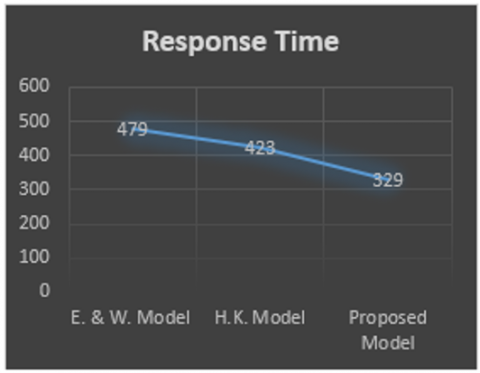Figure 3. Comparison of Response Time of Example 1

## 4.png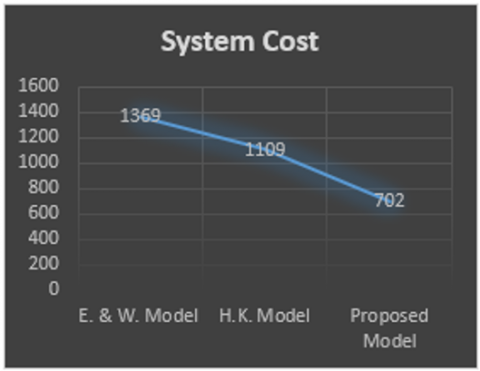Figure 4. Comparison of system cost of example 1

## 5.png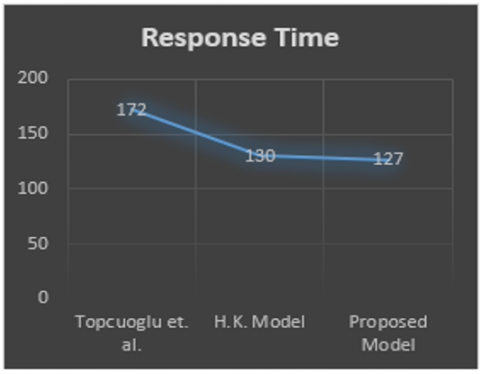Figure 5. Comparison of response time of example 2

## 6.png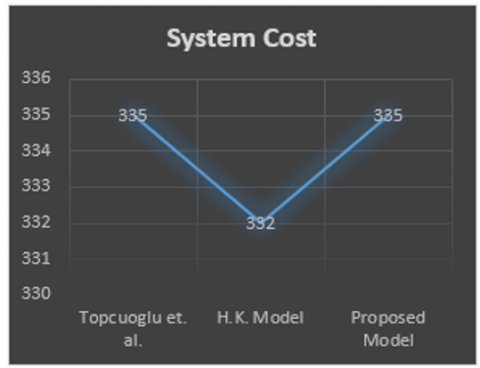Figure 6. Comparison of system cost of example 2

6. Conclusion and Future Scope

In this paper a task allocation problem has been formulated and shown in the form of mathematical model. Paper proposes a novel algorithm for allocating the tasks on different processors with the objective of minimum response time and system cost by taking Execution Time and Inter Task Communication Time into consideration. The algorithm uses fuzzy C – means clustering technique (to form the clusters) and Hungarian method (for allocation of clustered tasks to different processors). Paper illustrated two scenarios for testing the proposed algorithm which gives optimum OPRT and system cost. The model has potential to minimize the Overall Process Response Time and System Cost (for overlapped data) by assigning an approximate balanced load to the processors as per literature studied. The limitation of paper is that it has a restriction of using for static load balancing and task assignment. Moreover, in the proposed clustering technique the number of iterations increases if the termination criterion is lowered, thus making the technique lengthy. Although the model presented is efficient enough for unsupervised data but leaves a number of situations where further work can be done by making use of flexibility of the clustering technique used. In future it can be further explored by varying the values of the parameters, of the clustering technique used, for static and dynamic systems.

Acknowledgment

The author is extremely grateful to Dr. Jogendra Kumar, Dr. Garima Verma and Dr. Fateh Singh for their kind support, valuable suggestions, comments and help.

References

 Waraich, S.S. (2008). Classification of Dynamic Load Balancing strategies in a network of workstations. Fifth International Conference on Information Technology, New Generations, Las Vegas, NV, USA, pp. 1263-1265. https://doi.org/10.1109/ITNG.2008.166

 Huang, M.C., Hosseini, S.H., Vairaven, K. (2003). A Receiver-Initiated load balancing method in computer networks using fuzzy logic control. GLOBECOM '03. IEEE Global Telecommunications Conference (IEEE Cat. No.03CH37489), San Francisco, CA, USA, pp. 4028-4033. https://doi.org/10.1109/GLOCOM.2003.1258985

 Ahn, H.C., Youn, H.Y., Jeon, K.Y., Lee, K.S. (2007). Dynamic load balancing for large scale distributed system with intelligent fuzzy controller. IEEE International Conference on Information Reuse and Integration, Las Vegas, IL, USA, pp. 576-581. https://doi.org/10.1109/IRI.2007.4296682

 Zomaya, A.Y., Teh., Y.H. (2001). Observations on using Genetic algorithms for dynamic load balancing. IEEE Transaction on Parallel and Distributed Systems, 12(9): 899-911. https://doi.org/10.1109/71.954620

 Sriramdas, V., Chaurvedi, S.K., Gargama, H. (2014). Fuzzy arithmetic based reliability allocation approach during early design & development. Expert Systems with Applications, 41(7): 3444-3449. https://doi.org/10.1016/j.eswa.2013.10.048

 Neelkantan, P., Sreekanth, S. (2016). Task allocation in distributed systems. Indian Journal of Science & Technology, 9(31): 1-10. http://dx.doi.org/10.17485/ijst/2016/v9i31/89615

 Attiya, G., Hamam, Y. (2006). Task Allocation for maximizing reliability of distributed systems: A simulated annealing approach. Journal of Parallel and Distributed Computing, 66(10): 1259-1266. http://dx.doi.org/10.1016/j.jpdc.2006.06.006

 Kumar, H. (2015). A Heiristic model for task scheduling in heterogeneous distributed real time system under fuzzy environment. International Journal of Computer Applications, 111(2): 35-43.

 Hamed, A.Y. (2012). Task allocation for maximizing reliability of distributed computing systems using genetic algorithm. International Journal of Computer Networks and Wireless Communications, 2(5): 560-569.

 Topcuoglu, H., Hariri, S., Wu, M.Y. (2002). Performance effective & low complexity task scheduling for heterogeneous computing. IEEE Transactions on Parallel and Distributed Computing, 13(3): 260-274. http://dx.doi.org/10.1109/71.993206

 Falta, G.D., Blasa, F., Cafiero, S., Fortina, G. (2013). Fault tolerant decentralized k-means clustering for asynchronous large scale network. Journal of Parallel and Distributed Computing, 3(3): 317-329. https://doi.org/10.1016/j.jpdc.2012.09.009

 Akbari, M., Rashidi, H. (2016). A multi objective scheduling algorithm based on cuckoo optimization for task allocation problem at compile time in heterogeneous systems. Expert Systems with Applications, 60: 234-248. https://doi.org/10.1016/j.eswa.2016.05.014

 Bahmani, A., Mueller, F. (2016). Efficient clustering for ultra scale application tracing. Journal of Parallel & Distributed Computing, 98: 25-39. https://doi.org/10.1016/j.jpdc.2016.08.001

 Vidyarthi, D.P., Tripathi, A.K. (2001). Maximizing reliability of distributed computing systems with task allocation using simple genetic algorithm. J. System Architecture, 47(6): 549-554. https://doi.org/10.1016/S1383-7621(01)00013-3

 Chu, W.W., Holloway, I.J., Lan, M.T., Efe, K. (1980). Task allocation in distributed data processing. Journal Computer, 13(11): 57-69. http://dx.doi.org/10.1109/MC.1980.1653419

 Kumar, H., Chauhan, N.K., Yadav, P.K. (2018). A high performance model of task allocation in distributed computing system using k-means clustering technique. International Journal of Distributed Systems & Technologies, 9(3): 1-23. https://doi.org/10.4018/IJDST.2018070101

 Srinivasan, A., Geetharamani, G. (2013). Method for solving fuzzy assignment problem. Applied Mathematical Sciences, 7(113): 5607-5619. http://dx.doi.org/10.12988/ams.2013.37381

 Qinma, K., Hong, H., Jun, W. (2013). An effective iterated greedy algorithm for reliability-oriented task allocation in distributed computing systems. Journal of Parallel and Distributed Computing, 73(8): 1106-1115. https://doi.org/10.1016/j.jpdc.2013.03.008

 Rehman, S., Javaid, N., Rasheed, S., Hassan, K., Zafar, F., Naeem, M. (2018). Min-min scheduling algorithm for efficient resource distribution using cloud and fog in smart buildings. Proceedings of 13th International conference on Broadband and Wireless Computing Communication and Applications, pp. 15-27. http://dx.doi.org/10.1007/978-3-030-02613-4_2

 Jang, S.H., Kim, T.Y., Kim, J.K., Lee, J.S. (2012). The study of genetic algorithm-based task scheduling for cloud computing. International Journal of Control and Automation, 5(4): 157-162.